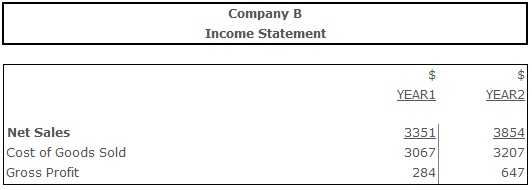# Gross Profit Margin

Gross Profit Margin – a profitability ratio measuring the amount of firm’s gross profit (revenue minus cost of goods sold) per dollar of sales.

## Formula(s):

Gross Profit Margin = Gross Profit ÷ Net Sales

## Example:Gross Profit Margin (Year 1) = 284 ÷ 3351= 0,11

Gross Profit Margin (Year 2) = 647 ÷ 3854 = 0,16

## Conclusion:

The increasing trend of the gross profit margin indicates the improving ability of an enterprise to earn gross profit. While in year 1 company was earning \$0,11 of the gross profit per dollar of sales, in year 2 this amount increased to \$0,16 per sales dollar.